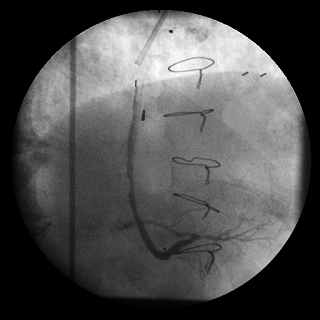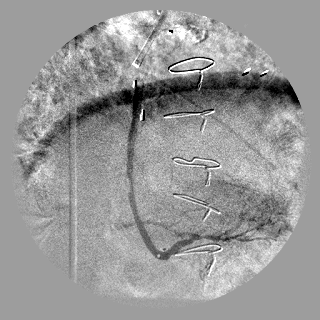﻿ DigitalSubtractCommand Class | Leadtools.ImageProcessing.Core | Raster, Medical, Document Help
←Select platform
In This Topic ▼

# DigitalSubtractCommand Class

Summary
Subtracts the live image from the mask image to show the differences between the two images.
Syntax
C#
Objective-C
C++/CLI
Java
Python
``public class DigitalSubtractCommand : RasterCommand ``
``@interface LTDigitalSubtractCommand : LTRasterCommand ``
````public class DigitalSubtractCommand `
`    extends RasterCommand ````
``public ref class DigitalSubtractCommand : public RasterCommand   ``
``class DigitalSubtractCommand(RasterCommand): ``
Remarks

This command is available in the Medical and above toolkits.

• Performs a digital subtraction between two images. The resulting image may be enhanced using the Flags options. Digital subtraction is mainly used to show the differences or the changes between two images. For the process to be meaningful both images should be related to each other. Digital Subtraction Angiography (DSA) is a practical example of digital subtraction.
• Digital Subtraction Angiography (DSA) and Quality Control (QC) are both major fields that use image subtraction. An example of DSA is explained below.
• DSA is a technology used for the visualization of blood vessels in the human body. A mask image is obtained using X-ray film of the body part of interest. Then vessels of that part are injected using a contrast medium. A sequence of images is taken after the injection. These are called live images. After applying subtraction between the mask and live images the resulting images are images that show the passage of the contrast medium in the vessels.
• Both the affected image and MaskImage should have the same bits per pixel, number of channels and image dimensions; otherwise the method will return an error code.
• If the DigitalSubtractCommandFlags.ContrastEnhancement flag is set then the contrast of the subtracted image will be enhanced.
• If the DigitalSubtractCommandFlags.OptimizeRange flag is set then the tonal range of the subtracted image will be optimized. That is, the image intensity will be distributed among the full image intensity range.
• This class supports 12 and 16-bit grayscale and 48 and 64-bit color images. Support for 12 and 16-bit grayscale and 48 and 64-bit color images is available only in the Document/Medical toolkits.
• This command does not support 32-bit grayscale images.
• All images must be signed or unsigned.

Digital Subtraction Angiography Function - BeforeDigital Subtraction Angiography Function - AfterView additional platform support for this Digital Subtraction Angiography function.

Example

Run the DigitalSubtractCommand on an image.

C#
````using Leadtools; `
`using Leadtools.Codecs; `
`using Leadtools.ImageProcessing.Core; `
` `
`public void DigitalSubtractCommandExample() `
`{ `
`   // Load an image `
`   RasterCodecs codecs = new RasterCodecs(); `
`   codecs.ThrowExceptionsOnInvalidImages = true; `
` `
`   RasterImage image = codecs.Load(Path.Combine(LEAD_VARS.ImagesDir, "DigitalSubtractionSource.j2k")); `
` `
`   // Prepare the command `
`   RasterImage MaskImage; `
`   MaskImage = codecs.Load(Path.Combine(LEAD_VARS.ImagesDir, "DigitalSubtractionMask.j2k"), 0, CodecsLoadByteOrder.Bgr, 1, 1); `
` `
`   DigitalSubtractCommand command = new DigitalSubtractCommand(); `
`   command.Flags = DigitalSubtractCommandFlags.ContrastEnhancement; `
`   command.MaskImage = MaskImage; `
`   command.Run(image); `
` `
`   codecs.Save(image, Path.Combine(LEAD_VARS.ImagesDir, "Result.jpg"), RasterImageFormat.Jpeg411, image.BitsPerPixel); `
`} `
` `
`static class LEAD_VARS `
`{ `
`   public const string ImagesDir = @"C:\LEADTOOLS22\Resources\Images"; `
`} ````
Requirements
Help Version 22.0.2023.3.31